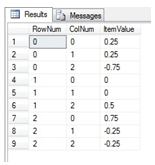# SQL Server MINVERSE function

MINVERSEN
Updated: 17 August 2010
Use MINVERSEN to calculate the matrix inverse of a square (N x N) array.

For matrices not in normalized form, use the MINVERSE function.

Syntax
SELECT * FROM [wctMath].[wct].[MINVERSEN] (
<@Matrix_TableName, nvarchar(4000),>
,<@Matrix_Key1ColumnName, nvarchar(4000),>
,<@Matrix_Key2ColumnName, nvarchar(4000),>
,<@Matrix_DataColumnName, nvarchar(4000),>
,<@Matrix_GroupedColumnName, nvarchar(4000),>
,<@Matrix_GroupedColumnValue, sql_variant,>)
Arguments
@Matrix_TableName
the name, as text, of the table or view that contains the values in the square (N x N) array to be used in the MINVERSEN calculation.
@Matrix_Key1ColumnName
the name, as text, of the column in the table or view specified by @Matrix_TableName that contains the ‘row number’ value used in the array.
@Matrix _Key2ColumnName
the name, as text, of the column in the table or view specified by @Matrix _TableName that contains the ‘column number’ value used in the array.
@Matrix _DataColumnName
The name, as text, of the column in the table or view specified by @Matrix _TableName that contains the matrix values to be used in the product. Data returned from the @Matrix_DataColumnName must be of the type float or of a type that implicitly converts to float.
@Matrix_GroupedColumnName
the name, as text, of the column in the table or view specified by @Matrix_TableName which will be used for grouping the results.
@Matrix_GroupedColumnValue
the column value to do the grouping on.
Return Types
TABLE (
[RowNum] [int] NULL,
[ColNum] [int] NULL,
[ItemValue] [float] NULL
Remarks
·         The number of columns in the array must equal the number of rows in the array.
·         If the matrix determinant is equal to zero an error will be returned.
·         Use the MINVERSEN_q function for more complex queries or to pass the matrices directly into the function without having to store them in a table.
·         Use MINVERSE for a table not in normal form.
·         If the array contains NULL, then NULL will be returned.
·         The function returns an error if the array contains a non-numeric value.
Examples

CREATE TABLE #i (
MatrixID    varchar(5),
rowno       int,
colno       int,
val               float
)

INSERT INTO #i VALUES ('1A',0,0,1.0)
INSERT INTO #i VALUES ('1A',0,1,2.0)
INSERT INTO #i VALUES ('1A',0,2,1.0)
INSERT INTO #i VALUES ('1A',1,0,3.0)
INSERT INTO #i VALUES ('1A',1,1,4.0)
INSERT INTO #i VALUES ('1A',1,2,-1.0)
INSERT INTO #i VALUES ('1A',2,0,0.0)
INSERT INTO #i VALUES ('1A',2,1,2.0)
INSERT INTO #i VALUES ('1A',2,2,0.0)

SELECT C.*
FROM wct.MINVERSEN(
'#i'
,'rowno'
,'colno'
,'val'
,'MatrixID'
,'1A'
) C

This produces the following resultIf we wanted to return the results in matrix form, we can use the PIVOT function, though this requires knowing the number of columns returned by the function (which is equal to the number of rows).

SELECT ,,
FROM (
SELECT C.*
FROM wct.MINVERSEN(
'#i'
,'rowno'
,'colno'
,'val'
,'MatrixID'
,'1A'
) C
) M PIVOT (
MAX(ItemValue)
FOR colnum IN (,,)
) AS pvt
ORDER BY rownum

This produces the following resultCopyright 2008-2023 Westclintech LLC         Privacy Policy        Terms of Service